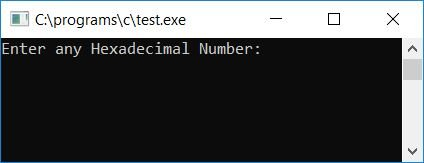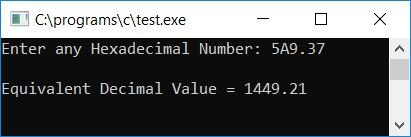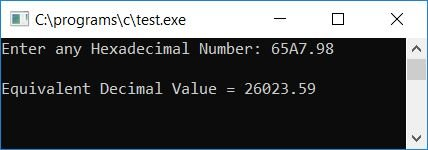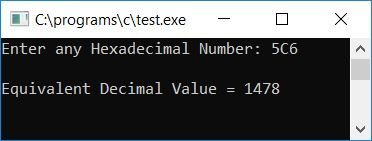# C Program to Convert Hexadecimal to Decimal

In this article, we will learn how to create a program in C that converts any given hexadecimal number entered by the user at run-time into its equivalent decimal value. We have also created a user-defined function that does the same job.

But before going through the program, if you are not aware of

• Decimal Number
• Hexadecimal to Decimal Conversion Process

Then follow the steps in the Hexadecimal to Decimal conversion process. Now let's move on to the program.

## Hexadecimal to Decimal in C

To convert a hexadecimal number to a decimal number in C programming, you have to ask the user to enter the hexadecimal number to convert it into a decimal number, and then display its equivalent value in decimal as an output. The question is, "Write a program in C that converts a hexadecimal number to a decimal." The answer to this question is:

```#include<stdio.h>
#include<conio.h>
#include<math.h>
int main()
{
int decnum=0, rem, i=0, len=0;
char hexnum;
scanf("%s", hexnum);
while(hexnum[i]!='\0')
{
len++;
i++;
}
len--;
i=0;
while(len>=0)
{
rem = hexnum[len];
if(rem>=48 && rem<=57)
rem = rem-48;
else if(rem>=65 && rem<=70)
rem = rem-55;
else if(rem>=97 && rem<=102)
rem = rem-87;
else
{
printf("\nYou've entered an invalid Hexadecimal digit");
getch();
return 0;
}
decnum = decnum + (rem*pow(16, i));
len--;
i++;
}
printf("\nEquivalent Decimal Value = %d", decnum);
getch();
return 0;
}```

As the above program was written in the Code::Blocks IDE, here is the first snapshot of the sample run:Now supply any hexadecimal number as input, say A67, and press the ENTER key to see its decimal equivalent, as shown in the second snapshot of the same sample run given below:#### Program Explained

• The entered hexadecimal number gets stored in a character array called hexnum.
• Using a while loop, count the length of the character array hexnum (the given hexadecimal number).
• After determining the length of the given hexadecimal number, decrement the length value (the variable len stores the length value) by one using either len-- or len=len-1. Because indexing in an array starts with 0.
• Initialize i with 0, because we are going to use i as an exponent of 16.
• Now create a while loop that runs from the last index to the first (the 0th index) of the given character array or hexnum.
• Therefore, at first run, the condition of the while loop len>=0 or 2>=0 (as the length of the given hexadecimal number 5A9 is 3, and after decrementing its value by 1, we have a len variable that holds 2 as its value) evaluates to true, therefore program flow goes inside the loop, and hexnum[len] or hexnum or 9 gets initialized to rem.
• As hexnum is a character and rem is an integer type variable, using rem = hexnum, the ASCII code of hexnum or 9 gets initialized to rem.
• And as we all know, the ASCII code for 0-9 is 48-57, and the ASCII code for A-F (which corresponds to hex digits 10-15) is 65-70.
• Therefore, we have checked and initialized the corresponding decimal value of a given hexadecimal digit using its ASCII code. That is, if hexnum holds 9, and the ASCII code for 9 is 56, then rem-48, 57-48, or 9 gets initialized to rem.
• After that, decnum + (rem*pow(16, i)) (decnum was initially set to 0 at the beginning of the program) or 0 + (9*pow(16, 0)) or 0 + 9*1 or 9 is assigned to decnum.
• The value of len gets decremented and becomes 1. And the value of i gets incremented and becomes 1.
• Program flow returns to the while loop condition, len>=0 or 1>=0 evaluates to true, and program flow again goes inside the loop.
• Carrying out a similar operation. That is, hexnum[len] or hexnum or A is set to rem. As the ASCII code for A is 65, therefore, rem-55 or 65-55 or 10 (10 represents A in hexadecimal) gets initialized to rem
• Then decnum + (rem*pow(16, i)) or 9 + (10*161) or 9+160 or 169 is set to decnum.
• The value of len gets decremented, and the value of i gets incremented.
• And program flow again goes back to the condition of the loop and does the same operation as told in the above steps.
• After exiting the while loop, we have the decnum variable, which holds the equivalent decimal value of the given hexadecimal number.
• Therefore, print the value of the decnum variable as an output.

### What if hexadecimal input contains decimals?

The program given above is only correct when the user supplies any hexadecimal number as input (without any decimal point). However, if he/she enters any hexadecimal number that includes a point (decimal point), you must replace the above program with the one below. The question is, "Write a program in C that converts hexadecimal (including the decimal point) to decimal." The answer to this question is:

```#include<stdio.h>
#include<conio.h>
#include<math.h>
int main()
{
int decnum=0, decnum1=0, rem, i=0, len=0, lenTemp, dotPos=0;
float decnum2=0;
char hexnum;
scanf("%s", hexnum);
while(hexnum[i]!='\0')
{
if(hexnum[i]=='.')
dotPos = i;
len++;
i++;
}
len--;
i=0;
if(dotPos==0)
{
while(len>=0)
{
rem = hexnum[len];
if(rem>=48 && rem<=57)
rem = rem-48;
else if(rem>=65 && rem<=70)
rem = rem-55;
else if(rem>=97 && rem<=102)
rem = rem-87;
else
{
printf("\nYou've entered an invalid Hexadecimal digit");
getch();
return 0;
}
decnum = decnum + (rem*pow(16, i));
len--;
i++;
}
printf("\nEquivalent Decimal Value = %d", decnum);
}
else
{
lenTemp = dotPos-1;
while(lenTemp>=0)
{
rem = hexnum[lenTemp];
if(rem>=48 && rem<=57)
rem = rem-48;
else if(rem>=65 && rem<=70)
rem = rem-55;
else if(rem>=97 && rem<=102)
rem = rem-87;
else
{
printf("\nYou've entered an invalid Hexadecimal digit");
getch();
return 0;
}
decnum1 = decnum1 + (rem*pow(16, i));
lenTemp--;
i++;
}
lenTemp = dotPos+1;
i=-1;
while(lenTemp<=len)
{
rem = hexnum[lenTemp];
if(rem>=48 && rem<=57)
rem = rem-48;
else if(rem>=65 && rem<=70)
rem = rem-55;
else if(rem>=97 && rem<=102)
rem = rem-87;
else
{
printf("\nYou've entered an invalid Hexadecimal digit");
getch();
return 0;
}
decnum2 = decnum2 + (rem*pow(16, i));
lenTemp++;
i--;
}
decnum2 = decnum1+decnum2;
printf("\nEquivalent Decimal Value = %0.2f", decnum2);
}
getch();
return 0;
}```

Here is a sample run of the above program. This is the final snapshot:#### Program Explained

• We used the variable dotPos to determine whether or not a decimal point is present within the given hexadecimal number.
• If present, then we have initialized 1 to the dotPos variable, and we check later whether the dotPos variable holds its previous value (zero) or not.
• If it holds, then we have to apply normal conversion rules as done in the first program given above; otherwise, we have to deal with different rules.
• In hexadecimal number input that contains a decimal point, we have used two-part
• One before and one after the decimal point.
• The before decimal part gets converted into its equivalent decimal value and initialized to decnum1, whereas the after decimal part gets converted into its equivalent decimal value and initialized to decnum2 (a floating point variable).
• Finally, add both variables together and initialize them to a floating-point variable, decnum2.
• and print its value as output.

### Hexadecimal to Decimal in C using a User-Defined Function

Now, as shown in the program below, let's create the same purpose program using a user-defined function called HexToDec(). The question is: "Write a program in C that converts hexadecimal to decimal using a user-defined function." The answer to this question is:

```#include<stdio.h>
#include<conio.h>
#include<math.h>
int HexToDecY(char hex[]);
float HexToDecN(char hex[]);
static int i, len, dotPos;
int main()
{
int decnumInt;
float decnumFlt;
char hexnum;
scanf("%s", hexnum);
while(hexnum[i]!='\0')
{
if(hexnum[i]=='.')
dotPos = i;
len++;
i++;
}
len--;
i=0;
if(dotPos==0)
{
decnumInt = HexToDecY(hexnum);
if(decnumInt!=0)
printf("\nEquivalent Decimal Value = %d", decnumInt);
}
else
{
decnumFlt = HexToDecN(hexnum);
if(decnumFlt!=0)
printf("\nEquivalent Decimal Value = %0.2f", decnumFlt);
}
getch();
return 0;
}
int HexToDecY(char hex)
{
int dec=0, rem;
while(len>=0)
{
rem = hex[len];
if(rem>=48 && rem<=57)
rem = rem-48;
else if(rem>=65 && rem<=70)
rem = rem-55;
else if(rem>=97 && rem<=102)
rem = rem-87;
else
{
printf("\nYou've entered an invalid Hexadecimal digit");
return 0;
}
dec = dec + (rem*pow(16, i));
len--;
i++;
}
return dec;
}
float HexToDecN(char hex)
{
int dec1=0, rem, lenTemp;
float dec2=0;
lenTemp = dotPos-1;
while(lenTemp>=0)
{
rem = hex[lenTemp];
if(rem>=48 && rem<=57)
rem = rem-48;
else if(rem>=65 && rem<=70)
rem = rem-55;
else if(rem>=97 && rem<=102)
rem = rem-87;
else
{
printf("\nYou've entered an invalid Hexadecimal digit");
return 0;
}
dec1 = dec1 + (rem*pow(16, i));
lenTemp--;
i++;
}
lenTemp = dotPos+1;
i=-1;
while(lenTemp<=len)
{
rem = hex[lenTemp];
if(rem>=48 && rem<=57)
rem = rem-48;
else if(rem>=65 && rem<=70)
rem = rem-55;
else if(rem>=97 && rem<=102)
rem = rem-87;
else
{
printf("\nYou've entered an invalid Hexadecimal digit");
return 0;
}
dec2 = dec2 + (rem*pow(16, i));
lenTemp++;
i--;
}
dec2 = dec1+dec2;
return dec2;
}```

Here is the final snapshot of the above program's sample run.#### Program Explained

• We have initialized the variables i, len, and dotPos as static and global variables.
• As a result, all three variables retain their previous values and are known throughout the program.
• We have not initialized 0 to all three static variables because static variables automatically hold 0 as their initial value.
• We have used two functions named HexToDecY() and HexToDecN().
• The function HexToDecY() deals with hexadecimal input without a decimal point, and the function HexToDecN() deals with hexadecimal input with a decimal point.
• We have supplied 65A7.98 as a hexadecimal number input.
• It contains a decimal point, therefore the HexToDecN() function gets called and executed.
• And finally, it returns dec2 to the main() function, which contains the decimal equivalent of the given hexadecimal number 65A7.98.
• In the main() function, the value of dec2 gets initialized to the decnumFlt variable, and the value of it gets printed as output.

Now let's take another sample run; this time we have supplied a hexadecimal number without any decimal point, as shown in the sample run given below. Here is another final snapshot of the sample run:#### The same program in different languages

C Quiz

« Previous Program Next Program »